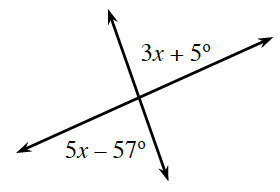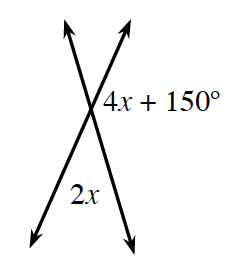### Home > GC > Chapter 2 > Lesson 2.1.2 > Problem2-18

2-18.

Examine the diagrams below. What is the geometric relationship between the labeled angles? What is the relationship of their measures? Then, use the relationship to write an equation and solve for $x$.

1.The labeled angles are vertical angles, so their measures are equal.

$3x+5º=5x-57º$

1.The labeled angles form a straight angle pair, so they are supplementary.